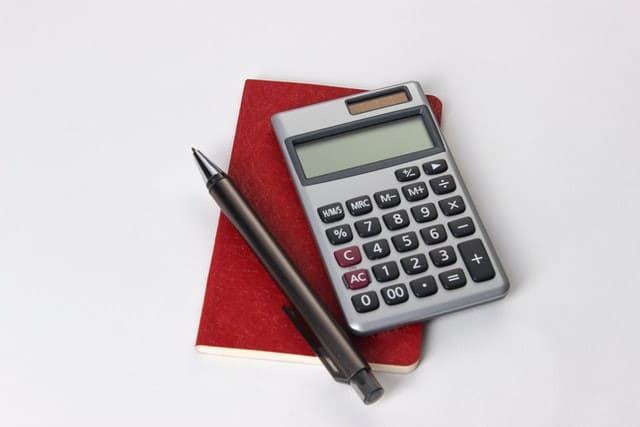# How to Use a Percent Error Calculator

By: Max Malak

7 min

0

10.04.2022Online calculators are specialized computer tools that let you answer problems online. They help you calculate the exact value without any human-like measurement errors. Such applications enable one to obtain a quick solution to a given job. It can be an easy addition to your homework process, which Studybay can further help you with.

There are several types of math calculators students can utilize, for instance, root calculator, lcm calculator, standard deviation calculator, etc. They can be used for tasks like calculating the absolute value, percent error equation, estimated value, function analysis, numerical methods, the absolute value of the difference, etc.

## What Is a Percentage Error Calculator?

The percentage error between an experimentally measured value and a real theoretical value may be calculated using the Percent Error Calculator.

The percent error is also known as the % error in mathematics, and it is defined as the percentage difference between the true values and observed values. In other words, a relative mistake multiplied by 100 is a percentage mistake. During the analysis phase, the percentage error is often determined to determine how significant our mistakes are.

## Percent Error Calculation

When analyzing data, the final output frequently differs from the real value. Mistakes can occur for a variety of causes, including human error, but they may also occur owing to approximations and limits of measuring instruments. Regardless, calculating the percentage inaccuracy might be useful in situations like these.

The absolute error, which is just the difference between the observed and actual value, is used to calculate percentage error. The relative error is then multiplied by 100 to get the percentage error, which is obtained by dividing the absolute error by the real value.

Take the following steps:

1. Subtract one value from the other to calculate the error, ignoring any minus signs.
2. Take the mistake and divide it by the precise value (we get a decimal number).
3. Calculate the percentage (by multiplying by 100 and adding a "percent "sign).

## Percent Error Formula

The percentage error is calculated using the following formula:

Percentage Error = [ (True value – observed value) / True value ] × 100

Here is an example of how to compute percent error.

We'll look at a basic example to help you better grasp what percent error is. Let's pretend we're trying to figure out how much of a difference there is between the measured and genuine sound speed.

Step 1. Find out what the true value of sound speed is. It's 343 m/s in the air at 20°C.

Step 2. Take an accurate reading. You observed that it was 330 m/s.

Step 3. Take the observed value and subtract it from the true value:

13 m/s= 343-330 m/s

Step 4. The absolute error is 13 m/s. Divide the correct number by the percent error to get the percent error:

percentage error= 13 / 343 *100%= 3.790 percent

Step 5. The relative error, often known as the percent error, equals 4.082 percent.

## Other Similar Terms

Percent error is one of several words that are used in this aspect. We've included a brief description of each of them below to ensure you don't get them mixed up.

### Standard Error

The standard error of a collection of data is a number that can be determined. It's a statistical word for the precision with which a sample accurately represents the entire population.

### Margin of Error

The confidence interval, on the other hand, is strongly connected to the margin of error. The confidence interval, on the other hand, is strongly connected to the margin of error.

## Using Percentage Change to Calculate Values

It might be handy to be able to determine real amounts based on percentage increases or decreases on occasion. In the media, instances of when this may be advantageous are widespread.

You could come across headlines like:

• The unemployment rate has dropped by 3%.
• Bonuses for bankers have been reduced by 39%.

These headlines offer a sense of a trend – where something is rising or declining–but there is rarely any actual data to back it up.

You can also opt for expert assistance in challenging math papers. At Studybay, you can get in contact with qualified professionals for research paper help and ultimately better grades.

# Percent Error Calculator

User ratings:

User ratings is 4.7 stars.Max Malak

Product Manager

Here at Studybay, I work as a Head of Affiliates in the marketing department. I studied Liberal Arts and took related classes at Tokyo Sophia University. I believe that challenges are what make my job fun and exciting. That's why I like completing complex, complicated, and even weird tasks and then sharing my experience with colleagues.